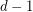Importance: Medium ✭✭
 Author(s): Rosenfeld, Moshe
 Subject: Graph Theory » Coloring » » Edge coloring
 Keywords: edge-coloring hypergraph Vizing
Conjecture   Letbe a simple-uniform hypergraph, and assume that every set ofpoints is contained in at mostedges. Then there exists an-edge-coloring so that any two edges which sharevertices have distinct colors.
Vizing's Theorem is equivalent to the above statement for. For higher dimensions, this problem looks difficult since the main tool used in the proof of Vizing's theorem (Kempe chains) do not appear to work.